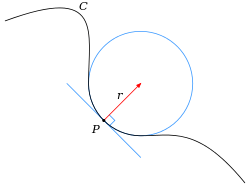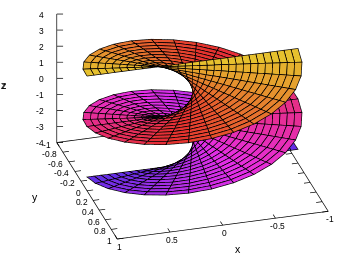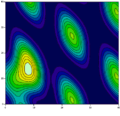# Multivariable calculus

Multivariable calculus (also known as multivariate calculus) is the extension of calculus in one variable to calculus with functions of several variables: the differentiation and integration of functions involving multiple variables, rather than just one.

## Typical operations

### Limits and continuity

A study of limits and continuity in multivariable calculus yields many counter-intuitive results not demonstrated by single-variable functions.:19–22 For example, there are scalar functions of two variables with points in their domain which give a particular limit when approached along any arbitrary line, yet give a different limit when approached along a parabola. For example, the functionapproaches zero along any line through the origin. However, when the origin is approached along a parabola, it has a limit of 0.5. Since taking different paths toward the same point yields different values for the limit, the limit does not exist.

Continuity in each argument is not sufficient for multivariate continuity::17–19 For instance, in the case of a real-valued function with two real-valued parameters,, continuity ofinfor fixedand continuity ofinfor fixeddoes not imply continuity of. As an example, considerIt is easy to verify that all real-valued functions (with one real-valued argument) that are given byare continuous in(for any fixed). Similarly, allare continuous asis symmetric with regards toand. However,itself is not continuous as can be seen by considering the sequence(for natural) which should converge toifwas continuous. However,Thus, function is not continuous at.

### Partial differentiation

Main article: Partial derivative

The partial derivative generalizes the notion of the derivative to higher dimensions. A partial derivative of a multivariable function is a derivative with respect to one variable with all other variables held constant.:26ff

Partial derivatives may be combined in interesting ways to create more complicated expressions of the derivative. In vector calculus, the del operator () is used to define the concepts of gradient, divergence, and curl in terms of partial derivatives. A matrix of partial derivatives, the Jacobian matrix, may be used to represent the derivative of a function between two spaces of arbitrary dimension. The derivative can thus be understood as a linear transformation which directly varies from point to point in the domain of the function.

Differential equations containing partial derivatives are called partial differential equations or PDEs. These equations are generally more difficult to solve than ordinary differential equations, which contain derivatives with respect to only one variable.:654ff

### Multiple integration

Main article: Multiple integral

The multiple integral expands the concept of the integral to functions of any number of variables. Double and triple integrals may be used to calculate areas and volumes of regions in the plane and in space. Fubini's theorem guarantees that a multiple integral may be evaluated as a repeated integral or iterated integral as long as the integrand is continuous throughout the domain of integration.:367ff

The surface integral and the line integral are used to integrate over curved manifolds such as surfaces and curves.

### Fundamental theorem of calculus in multiple dimensions

In single-variable calculus, the fundamental theorem of calculus establishes a link between the derivative and the integral. The link between the derivative and the integral in multivariable calculus is embodied by the integral theorems of vector calculus::543ff

In a more advanced study of multivariable calculus, it is seen that these four theorems are specific incarnations of a more general theorem, the generalized Stokes' theorem, which applies to the integration of differential forms over manifolds.

## Applications and uses

Techniques of multivariable calculus are used to study many objects of interest in the material world. In particular,

Domain/Codomain Applicable techniques
CurvesLengths of curves, line integrals, and curvature.
SurfacesAreas of surfaces, surface integrals, flux through surfaces, and curvature.
Scalar fieldsMaxima and minima, Lagrange multipliers, directional derivatives.
Vector fieldsAny of the operations of vector calculus including gradient, divergence, and curl.

Multivariable calculus can be applied to analyze deterministic systems that have multiple degrees of freedom. Functions with independent variables corresponding to each of the degrees of freedom are often used to model these systems, and multivariable calculus provides tools for characterizing the system dynamics.

Multivariate calculus is used in the optimal control of continuous time dynamic systems. It is used in regression analysis to derive formulas for estimating relationships among various sets of empirical data.

Multivariable calculus is used in many fields of natural and social science and engineering to model and study high-dimensional systems that exhibit deterministic behavior. In economics, for example, consumer choice over a variety of goods, and producer choice over various inputs to use and outputs to produce, are modeled with multivariate calculus. Quantitative analysts in finance also often use multivariate calculus to predict future trends in the stock market.

Non-deterministic, or stochastic systems can be studied using a different kind of mathematics, such as stochastic calculus.Wikimedia Commons has media related to Multivariate calculus.